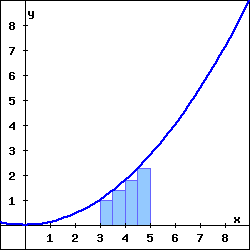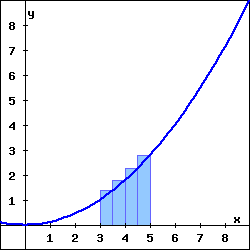Suppose $\displaystyle f(x) = \frac{x^2}{9}$.

(a) The rectangles in the graph on the left illustrate a left endpoint Riemann sum for $f(x)$ on the interval $3 \leq x \leq 5$. The value of this left endpoint Riemann sum is , and it is an the area of the region enclosed by $\displaystyle y = f(x)$, the x-axis, and the vertical lines $x = 3$ and $x = 5$.

(b) The rectangles in the graph on the right illustrate a right endpoint Riemann sum for $f(x)$ on the interval $3 \leq x \leq 5$. The value of this right endpoint Riemann sum is , and it is an the area of the region enclosed by $\displaystyle y = f(x)$, the x-axis, and the vertical lines $x = 3$ and $x = 5$.Left endpoint Riemann sum Right endpoint Riemann sum